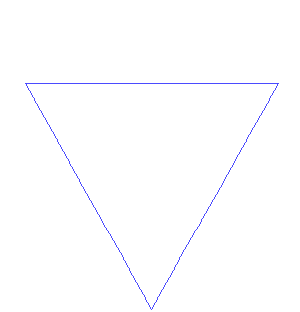# How much paint does it take to color in the Koch Snowflake?

Algebra Level 2What is the area enclosed by a Koch snowflake starting from an equilateral triangle with side length 1?

A. 1
B. $\frac{1}{2}$
C. $\frac{2\sqrt{3}}{5}$
D. $2 \frac{\sqrt{3}}{4}$
E. Area is infinite

×

Problem Loading...

Note Loading...

Set Loading...